# Capacitors and inductors in a circuit

#### Thrm

Joined Dec 31, 2021
24
Hi, I have been reading Lessons in Electric Circuits Volume II - AC
and they were talking about the concept of an electric pendulum and I did not get the concept of an inductor and capacitor in one circuit.

First I am very sorry for that awful long text, if you like, skip to the pictures. I just wanted to give you my "explanation" of capacitors and inductors because I clearly messed something up in the way they function. Thanks for the help tho!

First I will try to describe a capacitor and inductor in a circuit, because there clearly must be a mistake in my explanation:

Capacitor charging and the effect on the circuit (just power source and a capacitor):
- A capacitor is resisting changes in voltage and is dissipating power. This power gets stored inside the capacitor until the voltage equals that of the power source
- The effect of charging results in the total voltage is decreasing because the potential difference between the capacitor and the battery is getting less and less over time until its 0, thus current is decreasing as well because there is less "push" to move electrons

Capacitor discharging and the effect on the circuit (just power source and a capacitor):
- A capacitor is resisting changes in voltage and is supplying power. Voltage is decreasing because the electrons are leaving the negative charged plate trying to balance the potential difference, thus current is decreasing as well because there is less "push" to move electrons

Inductors charging and the effect on the circuit (just power source and a inductor):
- The inductor is resisting changes in current but as time moves one the inductor is resisting less current, thus greater voltage at each end of the inductor. Again, current and voltage increasing until fully charged

Inductors discharging and the effect on the circuit (just power source and a inductor):
- The inductor is resisting changes in current thus supplying a voltage to keep current constant. The inductor is discharging, thus less current through the inductor resulting in less voltage.
Again, current and voltage decreasing until fully discharged

Alright, my explaining is done (I think I messed some things up with the capacitor). Let get to the actual page in the book (122)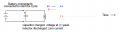( I agree)(I agree)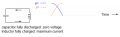(I agree)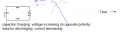(Where is the voltage coming from? Is it the voltage in the capacitor? Why is the current decreasing while voltage is increasing?)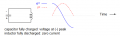(Why is current at 0 while the voltage is at its peak? Phase shifting? But how?)

And so on, but I am clearly missing the big picture its so frustrating I feel so stupid#### BobTPH

Joined Jun 5, 2013
4,907
Okay, you seem to get lost at the point where the voltage crosses zero. At that point what do you expect to happen?

Bob

#### crutschow

Joined Mar 14, 2008
29,800
Below is the LTspice simulation of your circuit with arbitrary value ideal components:

It's easier to understand the LC oscillation if you look at it as the system energy being transferred from the capacitor (1/2 CV²) to the inductor (1/2 LI²) and back in a sinusoidal fashion, similar to the way a mechanical pendulum mass transfers gravitational potential energy into inertial kinetic energy and back.
The inductor impedance to a change in current is the analog of the pendulum mass inertia, and the capacitance is the analog of the pendulum mass potential energy.

The simulation starts with the capacitor at 300V and all the energy stored in the capacitor (45 Joules calculated), and the inductor current (and energy) at zero (this would be similar to a pendulum being lifted to its maximum point before being released).
The capacitor voltage causes the inductor current to increase until the capacitor voltage is zero (at 50ms) and the inductor current is at its maximum value of ≈9.48A (the bottom of the pendulum cycle).
The inductor stored energy due to this current is now 45 Joules (calculated), the same as was in the capacitor at the start of the cycle.

This inductor inductance keeps the current flowing in the same direction and now starts charging the capacitor voltage with the opposite polarity until all the inductor energy is back in the capacitor
This back and forth cycle continues indefinitely here for the ideal components.
A real circuit would, of course, lose energy to the parasitic resistive losses with the oscillation amplitude reducing until the energy is depleted.

Since the maximum inductor current occurs at zero capacitor voltage, and the maximum capacitor voltage occurs at zero inductor current, the voltage is 90° out of phase with the current.

All make sense?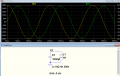#### Thrm

Joined Dec 31, 2021
24
Okay, you seem to get lost at the point where the voltage crosses zero. At that point what do you expect to happen?

Bob
Thank you for trying to help
I would say that either capacitor or inductor is at its final state of being charged and beginning to get discharged while the other one gets charged. But I don't know why and how...

#### Thrm

Joined Dec 31, 2021
24
Below is the LTspice simulation of your circuit with arbitrary value ideal components:

It's easier to understand the LC oscillation if you look at it as the system energy being transferred from the capacitor (1/2 CV²) to the inductor (1/2 LI²) and back in a sinusoidal fashion, similar to the way a mechanical pendulum mass transfers gravitational potential energy into inertial kinetic energy and back.
The inductor impedance to a change in current is the analog of the pendulum mass inertia, and the capacitance is the analog of the pendulum mass potential energy.

The simulation starts with the capacitor at 300V and all the energy stored in the capacitor (45 Joules calculated), and the inductor current (and energy) at zero (this would be similar to a pendulum being lifted to its maximum point before being released).
The capacitor voltage causes the inductor current to increase until the capacitor voltage is zero (at 50ms) and the inductor current is at its maximum value of ≈9.48A (the bottom of the pendulum cycle).
The inductor stored energy due to this current is now 45 Joules (calculated), the same as was in the capacitor at the start of the cycle.

This inductor inductance keeps the current flowing in the same direction and now starts charging the capacitor voltage with the opposite polarity until all the inductor energy is back in the capacitor
This back and forth cycle continues indefinitely here for the ideal components.
A real circuit would, of course, lose energy to the parasitic resistive losses with the oscillation amplitude reducing until the energy is depleted.

Since the maximum inductor current occurs at zero capacitor voltage, and the maximum capacitor voltage occurs at zero inductor current, the voltage is 90° out of phase with the current.

All make sense?

View attachment 258919
Thank you for your time and good explanation. It all makes sense now but one think has crossed my mind:

I understand that voltage is at 0 and current at maximum after the capacitor lost its voltage. But doesn't a inductor have voltage as well when fully charged? What role does it play when charging a capacitor because voltage starts at 0

#### MrChips

Joined Oct 2, 2009
26,113
Funny thing. I have taught electronics for over 40 years and have never examined the behavior of an LC circuit to a step function.
We always introduce RC and RL behavior to step functions. We also study RLC circuits to sinusoidal waves.

An LC circuit has a resonant frequency.
A step function has infinite frequencies. Hence an LC circuit will oscillate at its natural frequency thus the reference to an electric pendulum.

Think of a bell. If you hit a bell it will ring at its natural resonant frequency.

#### Thrm

Joined Dec 31, 2021
24
Funny thing. I have thought electronics for over 40 years and have never examined the behavior of an LC circuit to a step function.
We always introduce RC and RL behavior to step functions. We also study RLC circuits to sinusoidal waves.

An LC circuit has a resonant frequency.
A step function has infinite frequencies. Hence an LC circuit will oscillate at its natural frequency thus the reference to an electric pendulum.

Think of a bell. If you hit a bell it will ring at its natural resonant frequency.
Thank you for the answer, the analogy really helps but it brings up one question: As the current is at maximum, the inductor is generating a voltage and charging up the capacitor. Where does that fit into the analogy. The pendulum is at the bottom with full speed and zero height (the current is at maximum, the voltage at 0) but the inductor has voltage as it tries to continue the flow of electrons. Where on the graph can I find this state?

#### michael8

Joined Jan 11, 2015
287
An inductor doesn't have a voltage, it has a current. This current to a good approximate doesn't change
for small time intervals. The voltage you would see across the inductor is whatever it takes to be consistant
with the inductor current and the rest of the circuit.

•Thrm

#### crutschow

Joined Mar 14, 2008
29,800
. But doesn't a inductor have voltage as well when fully charged?
Not intrinsically.
What is "fully charged"?
Look at the equation for the inductor's energy. The energy is only a function of the current, with no mention of voltage.

Energy can be put into an inductor by applying a voltage to increase its current, but that voltage is not inherent to the inductor.
Then the inductance tries to keep that current flowing, the same way inertia tends to keep a moving mass moving.
Any voltage from that current is determined by the impedance in the circuit times the current.
In this case the impedance is the capacitive reactance.

So at the instant when the capacitor voltage is zero, the inductor current is at a maximum and charging the capacitor, with the voltage rising as the capacitor charges.

Last edited:

#### Thrm

Joined Dec 31, 2021
24
An inductor doesn't have a voltage, it has a current. This current to a good approximate doesn't change
for small time intervals. The voltage you would see across the inductor is whatever it takes to be consistant
with the inductor current and the rest of the circuit.
Thank you for your answer, this makes sense especially the analogy with the pendulum if you just think of an inductor of just having current

#### BobTPH

Joined Jun 5, 2013
4,907
The voltage across an inductor is:

V = L dI/dt

i.e. the voltage is proportional to the change in current. After the capacitor is fully discharged, the and the voltage is zero, the current must keep flowing because the change in current must be zero according to the above equation.

Since the current continues, it charges the capacitor with the opposite polarity. Now there is a negative voltage, and the current must decrease according to the above equation. The current keeps decreasing until it becomes zero, and now there is no current and the capacitor is charged in reverse to the same voltage as it gad originally.

Bob

#### Thrm

Joined Dec 31, 2021
24
The voltage across an inductor is:

V = L dI/dt

i.e. the voltage is proportional to the change in current. After the capacitor is fully discharged, the and the voltage is zero, the current must keep flowing because the change in current must be zero according to the above equation.

Since the current continues, it charges the capacitor with the opposite polarity. Now there is a negative voltage, and the current must decrease according to the above equation. The current keeps decreasing until it becomes zero, and now there is no current and the capacitor is charged in reverse to the same voltage as it gad originally.

Bob
Alright I think I got it now, it helped thinking about it in terms of equations, I kinda neglected that... I appreciate it!